# Returns the KL divergence, K(p1 || p2). : Math « Development Class « Java

Returns the KL divergence, K(p1 || p2).

```
/* Copyright (C) 2003 Univ. of Massachusetts Amherst, Computer Science Dept.
This file is part of "MALLET" (MAchine Learning for LanguagE Toolkit).
http://www.cs.umass.edu/~mccallum/mallet
This software is provided under the terms of the Common Public License,
information, see the file `LICENSE' included with this distribution. */

//package cc.mallet.util;

/**
*
*
* @author <a href="mailto:casutton@cs.umass.edu">Charles Sutton</a>
* @version \$Id: ArrayUtils.java,v 1.1 2007/10/22 21:37:40 mccallum Exp \$
*/
public class Util {
public static final double log2 = Math.log(2);
/**
* Returns the KL divergence, K(p1 || p2).
*
* The log is w.r.t. base 2. <p>
*
* *Note*: If any value in <tt>p2</tt> is <tt>0.0</tt> then the KL-divergence
* is <tt>infinite</tt>. Limin changes it to zero instead of infinite.
*
*/
public static double klDivergence(double[] p1, double[] p2) {

double klDiv = 0.0;

for (int i = 0; i < p1.length; ++i) {
if (p1[i] == 0) { continue; }
if (p2[i] == 0.0) { continue; } // Limin

klDiv += p1[i] * Math.log( p1[i] / p2[i] );
}

return klDiv / log2; // moved this division out of the loop -DM
}
}

```

### Related examples in the same category

 1 Absolute value 2 Find absolute value of float, int, double and long using Math.abs 3 Find ceiling value of a number using Math.ceil 4 Find exponential value of a number using Math.exp 5 Find floor value of a number using Math.floor 6 Find minimum of two numbers using Math.min 7 Find power using Math.pow 8 Find square root of a number using Math.sqrt 9 Find natural logarithm value of a number using Math.log 10 Find maximum of two numbers using Math.max 11 Get the power value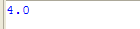12 Using the Math Trig Methods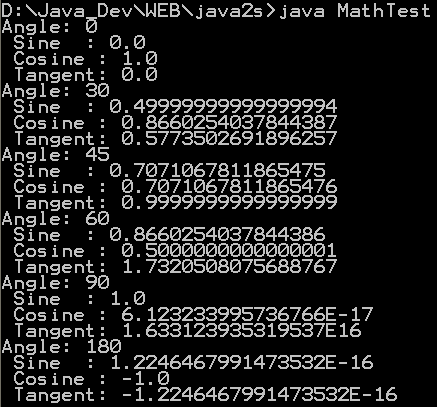13 Using BigDecimal for Precision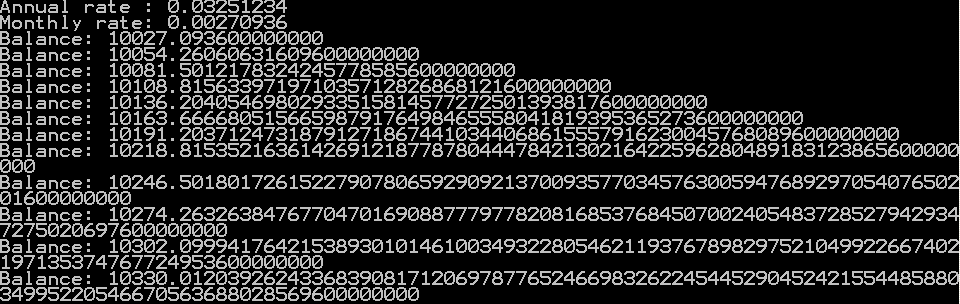14 Demonstrate our own version round()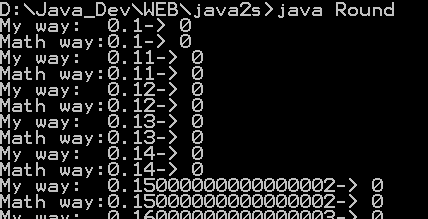15 Demonstrate a few of the Math functions for Trigonometry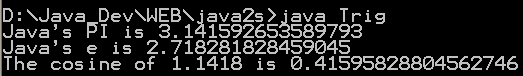16 Exponential Demo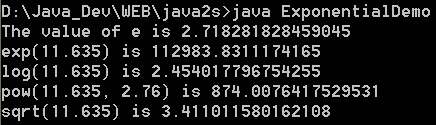17 Min Demo 18 Basic Math Demo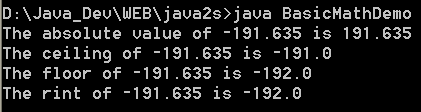19 Using strict math in applications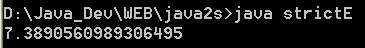20 Conversion between polar and rectangular coordinates 21 Using the pow() function 22 Using strict math at the method level 23 Calculating hyperbolic functions 24 Calculating trigonometric functions 25 Weighted floating-point comparisons 26 Solving right triangles 27 Applying the quadratic formula 28 Calculate the floor of the log, base 2 29 Greatest Common Divisor (GCD) of positive integer numbers 30 Least Common Multiple (LCM) of two strictly positive integer numbers 31 Moving Average 32 Make Exponention 33 Caclulate the factorial of N 34 Trigonometric Demo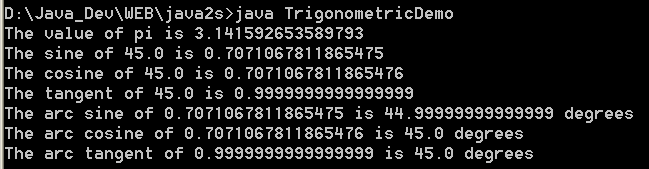35 Complex Number Demo 36 sqrt(a^2 + b^2) without under/overflow 37 Returns an integer hash code representing the given double array value. 38 Returns an integer hash code representing the given double value. 39 Returns n!. Shorthand for n Factorial, the product of the numbers 1,...,n as a double. 40 Returns n!. Shorthand for n Factorial, the product of the numbers 1,...,n. 41 Returns the hyperbolic sine of x. 42 Contains static definition for matrix math methods. 43 For a double precision value x, this method returns +1.0 if x >= 0 and -1.0 if x < 0. Returns NaN if x is NaN. 44 For a float value x, this method returns +1.0F if x >= 0 and -1.0F if x < 0. Returns NaN if x is NaN. 45 Normalize an angle in a 2&pi wide interval around a center value. 46 Normalizes an angle to a relative angle. 47 Normalizes an angle to an absolute angle 48 Normalizes an angle to be near an absolute angle 49 Returns the natural logarithm of n!. 50 Returns the least common multiple between two integer values. 51 Gets the greatest common divisor of the absolute value of two numbers 52 Matrix manipulation 53 Returns exact (http://mathworld.wolfram.com/BinomialCoefficient.html) Binomial Coefficient 54 Returns a double representation of the (http://mathworld.wolfram.com/BinomialCoefficient.html) Binomial Coefficient 55 Returns the natural log of the (http://mathworld.wolfram.com/BinomialCoefficient.html) Binomial Coefficient 56 Returns the hyperbolic cosine of x. 57 Math Utils 58 Implements the methods which are in the standard J2SE's Math class, but are not in in J2ME's. 59 Utility methods for mathematical problems. 60 A math utility class with static methods. 61 Computes the binomial coefficient "n over k" 62 Log Gamma 63 Log Beta 64 Beta 65 Gamma 66 Factorial 67 Computes p(x;n,p) where x~B(n,p) 68 Returns the sum of two doubles expressed in log space 69 sigmod 70 sigmod rev 71 Numbers that are closer than this are considered equal 72 Returns the sum of two doubles expressed in log space 73 Returns the difference of two doubles expressed in log space 74 Is Prime 75 Statistical functions on arrays of numbers, namely, the mean, variance, standard deviation, covariance, min and max 76 This class calculates the Factorial of a numbers passed into the program through command line arguments.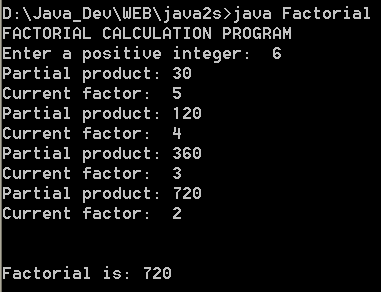77 Calculates the Greatest Common Divisor of two numbers passed into the program through command line arguments. 78 Variance: the square of the standard deviation. 79 Population Standard Deviation 80 Returns from a static prime table the least prime that is greater than or equal to a specified value.